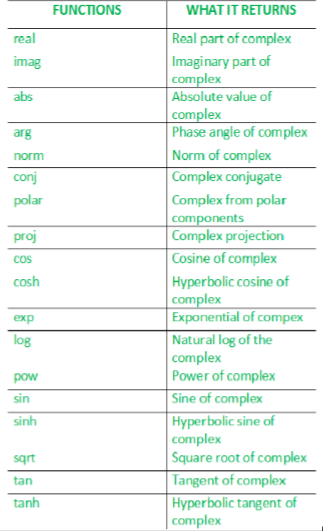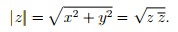Related Articles
Complex numbers in C++ | Set 1
• Last Updated : 06 Jul, 2017

The complex library implements the complex class to contain complex numbers in cartesian form and several functions and overloads to operate with them.• real() – It returns the real part of the complex number.
• imag() – It returns the imaginary part of the complex number.

 `// Program illustrating the use of real() and  ` `// imag() function ` `#include       ` ` `  `// for std::complex, std::real, std::imag ` `#include        ` `using` `namespace` `std; ` ` `  `// driver function ` `int` `main() ` `{     ` `  ``// defines the complex number: (10 + 2i) ` `  ``std::complex<``double``> mycomplex(10.0, 2.0); ` ` `  `  ``// prints the real part using the real function ` `  ``cout << ``"Real part: "` `<< real(mycomplex) << endl; ` `  ``cout << ``"Imaginary part: "` `<< imag(mycomplex) << endl; ` `  ``return` `0; ` `} `

Output:

```Real part: 10
Imaginary part: 2
```
• abs() – It returns the absolute of the complex number.
• arg() – It returns the argument of the complex number.

 `// Program illustrating the use of arg() and abs() ` `#include       ` ` `  `// for std::complex, std::abs, std::atg ` `#include   ` `using` `namespace` `std; ` ` `  `// driver function ` `int` `main () ` `{     ` `  ``// defines the complex number: (3.0+4.0i) ` `  ``std::complex<``double``> mycomplex (3.0, 4.0); ` ` `  `  ``// prints the absolute value of the complex number ` `  ``cout << ``"The absolute value of "` `<< mycomplex << ``" is: "``; ` `  ``cout << ``abs``(mycomplex) << endl; ` `   `  `  ``// prints the argument of the complex number ` `  ``cout << ``"The argument of "` `<< mycomplex << ``" is: "``; ` `  ``cout << arg(mycomplex) << endl; ` ` `  `  ``return` `0; ` `} `

Output:

```The absolute value of (3,4) is: 5
The argument of (3,4) is: 0.927295
```
• polar() – It constructs a complex number from magnitude and phase angle.

real = magnitude*cosine(phase angle)
imaginary = magnitude*sine(phase angle)

 `// Program illustrating the use of polar() ` `#include       ` ` `  `// std::complex, std::polar ` `#include ` `using` `namespace` `std; ` ` `  `// driver function ` `int` `main () ` `{ ` `  ``cout << ``"The complex whose magnitude is "` `<< 2.0; ` `  ``cout << ``" and phase angle is "` `<< 0.5; ` `   `  `  ``// use of polar() ` `  ``cout << ``" is "` `<< polar (2.0, 0.5) << endl; ` ` `  `  ``return` `0; ` `} `

Output:

```The complex whose magnitude is 2 and phase angle is 0.5 is (1.75517,0.958851)
```
• norm() – It is used to find the norm(absolute value) of the complex number. If z = x + iy is a complex number with real part x and imaginary part y, the complex conjugate of z is defined as z'(z bar) = x – iy, and the absolute value, also called the norm, of z is defined as :`// example to illustrate the use of norm() ` `#include       ` ` `  `// for std::complex, std::norm ` `#include   ` `using` `namespace` `std; ` ` `  `// driver function ` `int` `main () ` `{     ` `  ``// initializing the complex: (3.0+4.0i) ` `  ``std::complex<``double``> mycomplex (3.0, 4.0); ` ` `  `  ``// use of norm() ` `  ``cout << ``"The norm of "` `<< mycomplex << ``" is "`  `       ``<< norm(mycomplex) <

Output:

```The norm of (3,4) is 25.
```
• conj() – It returns the conjugate of the complex number x. The conjugate of a complex number (real,imag) is (real,-imag).

 `// Illustrating the use of conj() ` `#include   ` `using` `namespace` `std; ` ` `  `// std::complex, std::conj ` `#include        ` ` `  `// driver program ` `int` `main () ` `{ ` `  ``std::complex<``double``> mycomplex (10.0,2.0); ` ` `  `  ``cout << ``"The conjugate of "` `<< mycomplex << ``" is: "``; ` `   `  `  ``// use of conj() ` `  ``cout << conj(mycomplex) << endl; ` `  ``return` `0; ` `} `

Output:

``` The conjugate of (10,2) is (10,-2)
```
• proj() – It returns the projection of z(complex number) onto the Riemann sphere. The projection of z is z, except for complex infinities, which are mapped to the complex value with a real component of INFINITY and an imaginary component of 0.0 or -0.0 (where supported), depending on the sign of the imaginary component of z.

 `// Illustrating the use of proj() ` ` `  `#include ` `using` `namespace` `std; ` ` `  `// For std::complex, std::proj ` `#include ` `  `  `// driver program ` `int` `main() ` `{ ` `    ``std::complex<``double``> c1(1, 2); ` `    ``cout << ``"proj"` `<< c1 << ``" = "` `<< proj(c1) << endl; ` `  `  `    ``std::complex<``double``> c2(INFINITY, -1); ` `    ``cout << ``"proj"` `<< c2 << ``" = "` `<< proj(c2) << endl; ` `  `  `    ``std::complex<``double``> c3(0, -INFINITY); ` `    ``cout << ``"proj"` `<< c3 << ``" = "` `<< proj(c3) << endl; ` `} `

Output:

```proj(1,2) = (1,2)
proj(inf,-1) = (inf,-0)
proj(0,-inf) = (inf,-0)
```
• sqrt() – Returns the square root of x using the principal branch, whose cuts are along the negative real axis.

 `// Illustrating the use of sqrt() ` `#include ` `using` `namespace` `std; ` ` `  `// For std::ccomplex, stdc::sqrt ` `#include ` `  `  `// driver program ` `int` `main() ` `{     ` `    ``// use of sqrt() ` `    ``cout << ``"Square root of -4 is "` `         ``<< ``sqrt``(std::complex<``double``>(-4, 0)) << endl ` `         ``<< ``"Square root of (-4,-0), the other side of the cut, is "` `         ``<< ``sqrt``(std::complex<``double``>(-4, -0.0)) << endl; ` `} `

Output:

```Square root of -4 is (0,2)
Square root of (-4,-0), the other side of the cut, is (0,-2)
```

Next article: Complex numbers in C++ | Set 2

This article is contributed by Shambhavi Singh. If you like GeeksforGeeks and would like to contribute, you can also write an article using contribute.geeksforgeeks.org or mail your article to contribute@geeksforgeeks.org. See your article appearing on the GeeksforGeeks main page and help other Geeks.